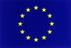## Visual Introduction to Bifurcations

Kulcsszavak: Dynamic systems, bifurcation, experimental study, Wolfram Mathematica
Absztrakt:
In discrete and continuous time models of life sciences, physics and engineering, it very often happens, that the difference/differential equation contains parameters and the value of these parameters are often only known approximately. In particular, they are generally determined by measurments which are not exact. For that reason, it is important to study the the behavior of the solutions and examine their dependence of parameters. This study leads to the bifurcation theory what is getting more and more important in most fields of engineering and sciences. Nevertheless, these theories are hardly included in standard university curricula.
Our lecture notes give an intuitive introduction with the help of dynamic demonstrations developed in Wolfram Mathematica. We consider elementary examples of both difference and differential equations presenting different types of bifurcation. During the whole treatment, we keep in mind the real didactic “contradiction” that the students do not or only partly have the required knowledge.

The development is co-financed by the European Union in the frame of the project IPA HU-SRB/1203/221/024: Non-Standard Forms of Teaching Mathematics and PhysicsSzükséges szoftverek:

Read and play: Wolfram CDF player
Full functionality: Wolfram Mathematica

Tartalomjegyzék: